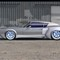Elite indicators :) - page 1440372

I don't (not with version 4_4 nor with version 4_5) so i do not know why are you getting that issue. Sorry ...
can it be hardware related? just a question are you calling the indi from an EA? because if i just put it on a chart i have no issues31

Can you please update this MACD_OsMA_4ColorH_2LVar_mtf for MT4 BUILD 1010 .. or fix it if the problem is not in the update? Thank you in advance.

Files:151720

fabiogeraci:
can it be hardware related? just a question are you calling the indi from an EA? because if i just put it on a chart i have no issues

Post your iCustom() call so that it can be revised372

Post your iCustom() call so that it can be revised

int IndiPeriod = 20;
int AveType = ma_ema; //from post in page 861
bool dSmoothed = false;

double tUPlevel = 0.05;
double tDownl = -0.05;
double Slen = 0;
double Sphase = 0;
bool color = false;
int interpMethod = 0; // for no interpolation

String TrendDirForce = "Trend direction and force index - smoother 4_4";151720

fabiogeraci:

int IndiPeriod = 20;
int AveType = ma_ema; //from post in page 861
bool dSmoothed = false;

double tUPlevel = 0.05;
double tDownl = -0.05;
double Slen = 0;
double Sphase = 0;
bool color = false;
int interpMethod = 0; // for no interpolation

String TrendDirForce = "Trend direction and force index - smoother 4_4";

Use it like this :

PS: your "color" variable can not have that name, I assumed that PriceType is correct (used 0 for testing) and TrendDirForce name is wrong (it should be "smoothed" not "smoother")372

Use it like this :

PS: your "color" variable can not have that name, I assumed that PriceType is correct (used 0 for testing) and TrendDirForce name is wrong (it should be "smoothed" not "smoother")
No Luck, how would i go about to code the basic buffer into a double into my EA?11

Is it possible to have atr slope for EA indicator?

cougar68:

Is it possible to have atr slope for ea indicator?

Thank you. (Maybe using "ATR ratio" indicator filtering out slope down and below a certain adjustable level.)151720

fabiogeraci:
No Luck, how would i go about to code the basic buffer into a double into my EA?

Sorry, but what does the luck have to do with it?

If you use the code I posted, there is no "too slow" message (tested and retested on every symbol I have and all is working exactly as it should). Here is the code I used for testing (the only thing I changed is to access buffer 7 not buffer 0, since in buffer 7 is the state of the "trend", while in buffer 0 you have the upper trigger value) and this code did not cause and error message (attaching the file too, just in case)

#property indicator_chart_window

enum enMaTypes
{
ma_ahr,     // Ahrens moving average
ma_alxma,   // Alexander moving average - ALXMA
ma_dema,    // Double exponential moving average - DEMA
ma_dsema,   // Double smoothed exponential moving average - DSEMA
ma_emas,    // Ema derivative - EMAD
ma_ema,     // Exponential moving average - EMA
ma_hull,    // Hull moving average - HMA
ma_ie2,     // IE/2
ma_ilinr,   // Integral of linear regression slope
ma_itl,     // Instantaneous trendline
ma_lagg,    // Laguerre filter
ma_linr,    // Linear regression value - LSMA
ma_lwma,    // Linear weighted moving average - LWMA
ma_mcg,     // McGinley Dynamic
ma_mcma,    // McNicholl ema
ma_nlma,    // Non lag moving average
ma_pwma,    // Parabolic weighted moving average - PWMA
ma_rmta,    // Recursive moving trendline - RMTA
ma_sma,     // Simple moving average - SMA
ma_sine,    // Sine weighted moving average
ma_smma,    // Smoothed moving average - SMMA
ma_smoo,    // Smoother
ma_ssm,     // Super smoother
ma_b3p,     // Three pole Ehlers Butterworth
ma_s3p,     // Three pole Ehlers smoother
ma_tma,     // Triangular moving average - TMA
ma_tema,    // Tripple exponential moving average - TEMA
ma_b2p,     // Two pole Ehlers Butterworth
ma_s2p,     // Two pole Ehlers smoother
ma_vema,    // Volume weighted ema - VEMA
ma_vwma,    // Volume weighted moving average - VWMA
ma_zldema,  // Zero lag dema
ma_zlma,    // Zero lag moving average
ma_zltema   // Zero lag tema
};

int IndiPeriod = 20;
int AveType = ma_ema; //from post in page 861
bool dSmoothed = false;

double tUPlevel = 0.05;
double tDownl = -0.05;
double Slen = 0;
double Sphase = 0;
bool tcolor = false;
int interpMethod = 0; // for no interpolation

string TrendDirForce = "Trend direction and force index - smoothed 4_4";

int init()   { return(0); }
int deinit() { return(0); }
int start()
{
int PriceType=0;
Comment(tdfValue);
return(0);
}
Files:
_test.mq4 3 kb151720

Same thing done as an expert - same results - no error message
Files:
_test.mq4 3 kb372

Thank you for the help, I have tried your code and yes it does work, but when i use the same call in EA i get that warning